# Class 11 Maths NCERT Solutions for Chapter 13 Limits and Derivatives Miscellaneous Exercise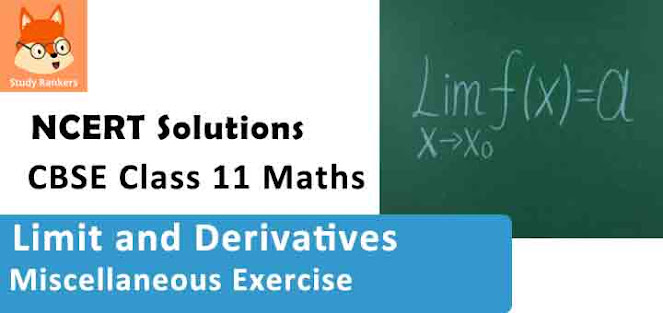### Limits and Derivatives Miscellaneous Exercise Solutions

1. Find the derivative of the following functions from first principle:

(i) –x
(ii) (–x)–1
(iii) sin (x + 1)
(iv) cos(x - π/8)

Solution

(i) Let f(x) = -x . Accordingly, f(x + h) = -(x + h)
By first principle,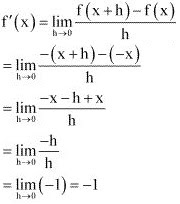(ii) Let f(x) = (-x)–1 = 1/-x = -1/x . Accordingly, f(x + h) = -1/(x + h)
By first principle,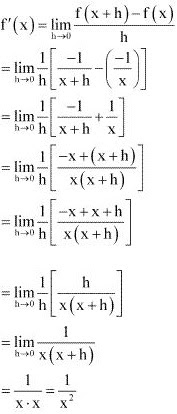(iii) Let f(x) = sin (x + 1). Accordingly, f(x + h) = sin(x + h+ 1)
By first principle,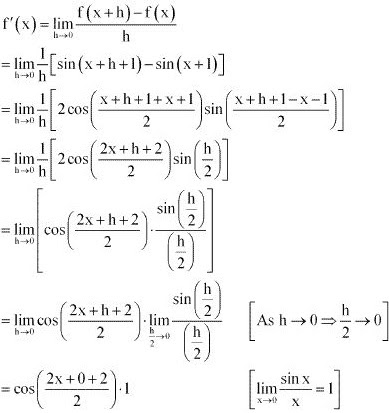= cos(x + 1)

(iv) Let f(x) = cos (x - π/8). Accordingly, f(x + h) = cos (x + h - π/8)
By first principle,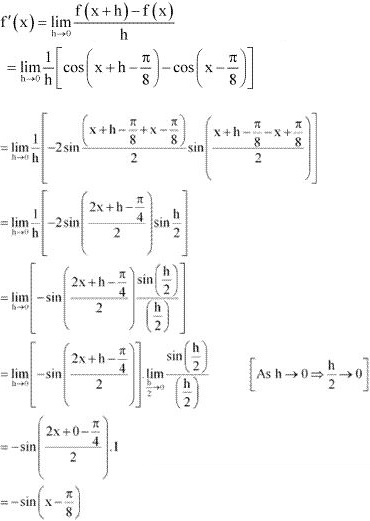2. Find the derivative of the following functions (it is to be understood that a, b, c, d, p, q, r and s are fixed non-zero constants and m and n are integers): (x + a)

Solution

Let f(x) = x + a. Accordingly, f(x + h) = x + h + a
By first principle,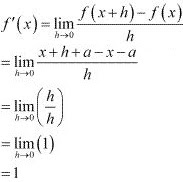3. Find the derivative of the following functions (it is to be understood that a, b, c, d, p, q, r and s are fixed non-zero constants and m and n are integers): (px + q)(r/s + s)

Solution

Let f(x) = (px + q)(r/s + s)
By Leibnitz product rule,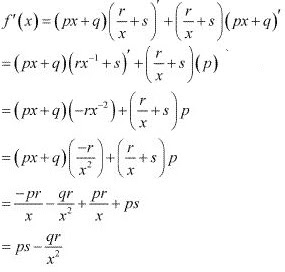4. Find the derivative of the following functions (it is to be understood that a, b, c, d, p, q, rand s are fixed non-zero constants and m and n are integers): (ax + b) (cx + d)2
Solution
Let f(x) = (ax + b)(cx + d)2
By Leibnitz product rule,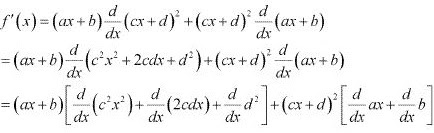= (ax + b)(2c2 x + 2cd) + (cx + d2)a
= 2c(ax + b)(cx + d) + a(cx + d)2

5. Find the derivative of the following functions (it is to be understood that a, b, c, d, p, q, rand s are fixed non-zero constants and m and n are integers):  (ax + b)/(cx + d).
Solution
Let f(x) = (ax+  b)/(cx + d)
By quotient rule,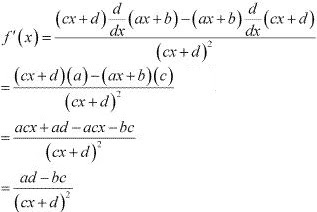6. Find the derivative of the following functions (it is to be understood that a, b, c, d, p, q, r and s are fixed non-zero constants and m and n are integers): (1 + 1/x)/(1 - 1/x).
Solution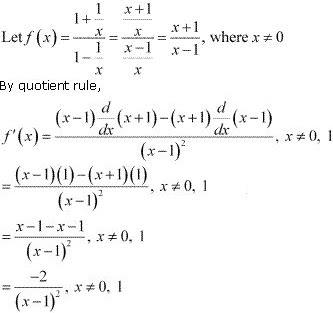7. Find the derivative of the following functions (it is to be understood that a, b, c, d, p, q, r and s are fixed non-zero constants and m and n are integers): 1/(ax2 + bx + c) .
Solution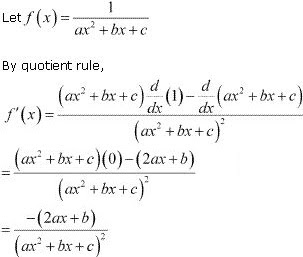8. Find the derivative of the following functions (it is to be understood that a, b, c, d, p, q, r and s are fixed non-zero constants and m and n are integers) : (ax + b)/(px2+ qx + r) .
Solution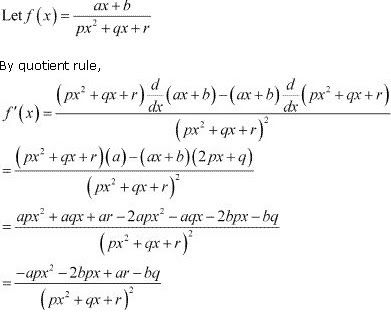9. Find the derivative of the following functions (it is to be understood that a, b, c, d, p, q, r and s are fixed non-zero constants and m and n are integers): (px2 + qx + r)/(ax + b)

Solution10. Find the derivative of the following functions (it is to be understood that a, b, c, d, p, q, rand s are fixed non-zero constants and m and n are integers) : a/x4 = (b/x2) + cos x
Solution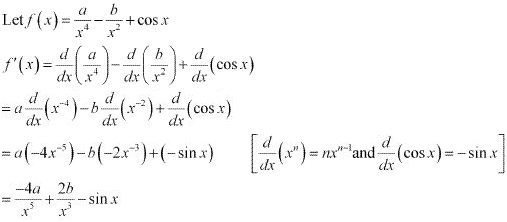11. Find the derivative of the following functions (it is to be understood that a, b, c, d, p, q, rand s are fixed non-zero constants and m and n are integers): 4√x – 2 .
Solution
Let f(x) = 4√x – 2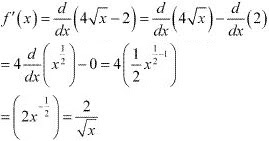12. Find the derivative of the following functions (it is to be understood that a, b, c, d, p, q, rand s are fixed non-zero constants and m and n are integers): (ax + b)n .
Solution
Let f(x) = (ax + b)n . Accordingly, f(x + h) = {a(x + h) + b}n = (ax + ah + b)n
By first principle,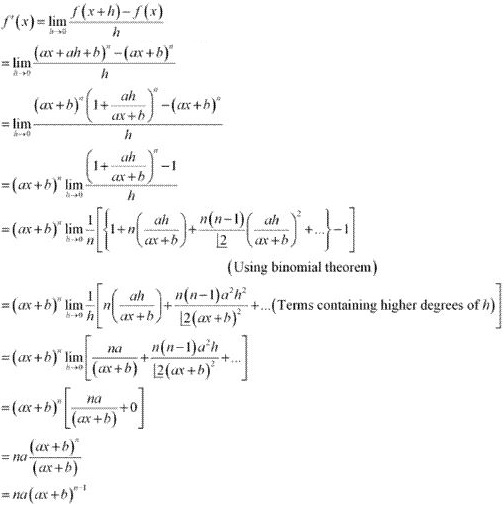13. Find the derivative of the following functions (it is to be understood that a, b, c, d, p, q, r and s are fixed non-zero constants and m and n are integers): (ax + b)n (cx + d)m
Solution
Let f(x) = (ax + b)n (cx + d)m
By Leibnitz product rule,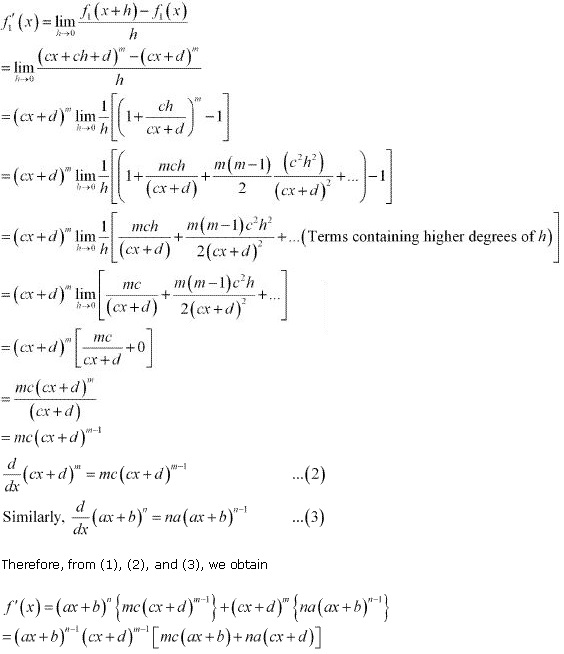14. Find the derivative of the following functions (it is to be understood that a, b, c, d, p, q, r and s are fixed non-zero constants and m and n are integers): sin (x + a)
Solution
Let f(x) = sin (x + a)
f(x + h) = sin (x +  h + a)
By first principle,15. Find the derivative of the following functions (it is to be understood that a, b, c, d, p, q, r and s are fixed non-zero constants and m and n are integers): cosec x cot x
Solution
Let f(x) = cosec x cot x
By Leibnitz product rule,
f'(x) = cosec x (cot x)' + cot x(cosec x)'  ...(1)
Let f1 (x) = cos x. Accordingly, f1 (x + h) = cot (x + h)
By first principle,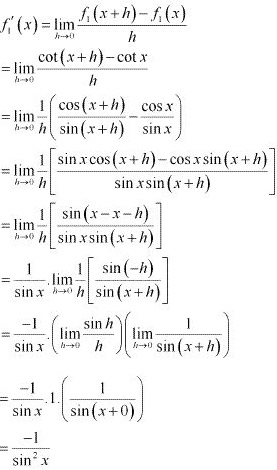= - cosec2 x
∴ (cot x)' = -cosec2 x ...(2)
Now, let f2 (x) = cosec x . Accordingly, f2 (x + h) = cosec (x + h)
By first principle,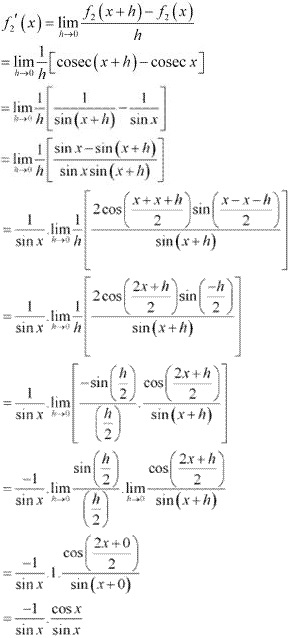= - cosec x . cot x
∴ (cosec x)' = -cosec x. cot x  ...(3)
From (1), (2), and (3), we obtain
f '(x) = cosec x(- cosec2 x) + cot x( -cosec x cot x)
= -cosec3 x - cot2 x cosec x

16. Find the derivative of the following functions (it is to be understood that a, b, c, d, p, q, r and s are fixed non-zero constants and m and n are integers):
Solution
Let f(x) = cos x/(1 + sin x)
By quotient rule,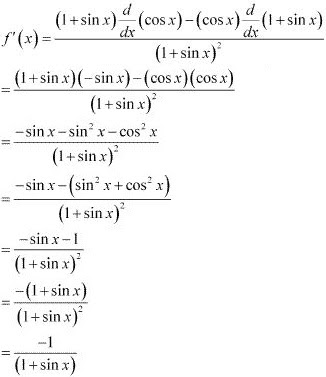17. Find the derivative of the following functions (it is to be understood that a, b, c, d, p, q, r and s are fixed non-zero constants and m and n are integers) :  (sin x + cos x)/(sin x - cos x)
Solution
Let f(x) = (sin x + cos x)/(sin x - cos x)
By quotient rule,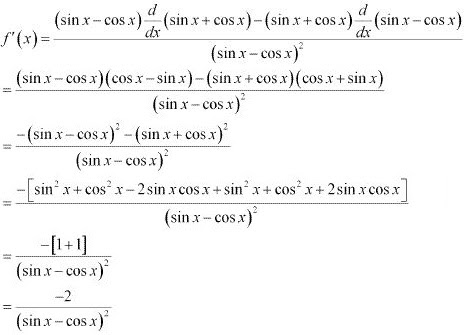18. Find the derivative of the following functions (it is to be understood that a, b, c, d, p, q, r and s are fixed non-zero constants and m and n are integers) : (sec x - 1)/(sec x + 1)
Solution
Let f(x) = (sec x - 1)/(sec x + 1)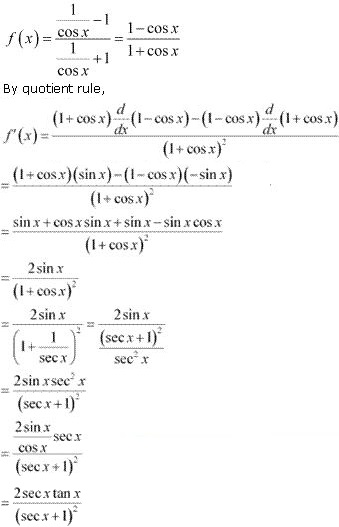19. Find the derivative of the following functions (it is to be understood that a, b, c, d, p, q, r and s are fixed non-zero constants and m and n are integers): sinn x
Solution
Let y = sinn  x.
Accordingly, for n = 1, y = sin x.
∴ dy/dx = cos x, i.e., (d/dx) sin x = cos x
For n = 2, y = sin2 x.
∴ dy/dx = (d/dx) (sin x sin x)
= (sin x)' sin x + sin x(sin x)'  [By Leibnitz product rule]
= cos x sin x + sin x cos x
= 2 sin x cos x  ...(1)
For n = 3, y = sin3 x .
∴ dy/dx = (d/dx)(sin x sin2 x)
= (sin x)' sin2 x + sin x(sin2 x)'  [By Leibnitz product rule]
= cos x sin2 x + 2 sin2 x cos x
= 3 sin2 x cos x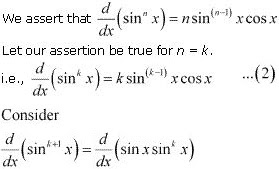= (sin x)' sink x + sin x(sink x)'   [By Leibnitz product rule]
= cos x sink x + sin x(k sin(k-1) x cos x)   [Using (2)]
= cos x sink x  + k sink x cos x
= (k + 1)sink x cos x
Thus, our assertion is true for n = k + 1
Hence, by mathematical induction , d/dx (sinn x) = n sin(n-1) x cos x

20. Find the derivative of the following functions (it is to be understood that a, b, c, d, p, q, r and s are fixed non-zero constants and m and n are integers): (a + b sinx)/(c + d cos x)
Solution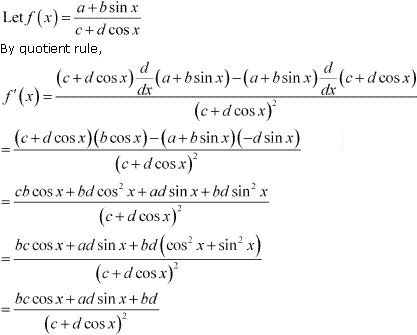21. Find the derivative of the following functions (it is to be understood that a, b, c, d, p, q, r and s are fixed non-zero constants and m and n are integers): [sin (x + a)]/cos x  .
Solution
Let f(x) = [sin (x + a)]/cos x
By quotient rule,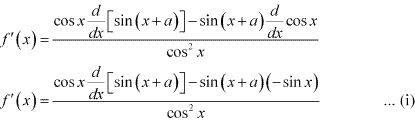Let g(x) = sin (x + a). Accordingly, g(x + h) = sin (x + h + a)
By first principle,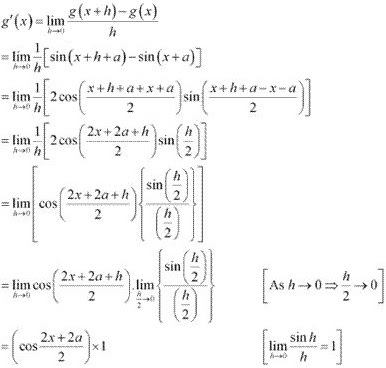= cos (x + a)  ...(ii)
From (i) and (ii), we obtain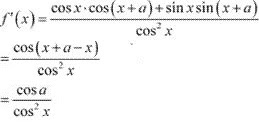22. Find the derivative of the following functions (it is to be understood that a, b, c, d, p, q, r and s are fixed non-zero constants and m and n are integers): x4 (5 sin x – 3 cos x)
Solution
Let f(x) = x4 (5 sin x – 3 cos x)
By product rule,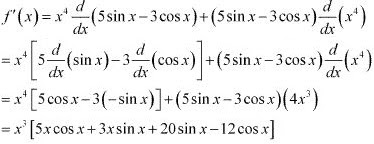23. Find the derivative of the following functions (it is to be understood that a, b, c, d, p, q, r and s are fixed non-zero constants and m and n are integers): (x2 + 1) cos x
Solution
Let f(x) = (x2 + 1) cos x
By product rule,= (x2 + 1)(- sin x) + cos x(2x)
= -x2 sin x - sin x + 2x cos x

24. Find the derivative of the following functions (it is to be understood that a, b, c, d, p, q, r and s are fixed non-zero constants and m and n are integers): (ax2 + sin x) (p + q cos x)
Solution
Let f(x) = (ax2 + sin x)(p + q cos x)
By product rule,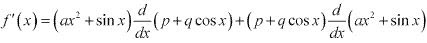= (ax2 + sin x)(-q sin x)  + (p + q cos x)(2ax + cos x)
= -q sin x (ax2 + sin x) + (p + q cos x) (2ax + cos x)

25. Find the derivative of the following functions (it is to be understood that a, b, c, d, p, q, r and s are fixed non-zero constants and m and n are integers): (x + cos x)(x - tan x)
Solution
Let f(x) = (x + cos x)(x - tan x)
By product rule,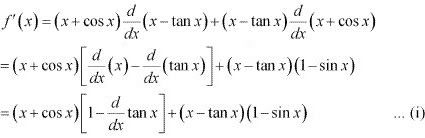Let g(x) = tan x. Accordingly, g(x + h) = tan (x + h)
By first principle,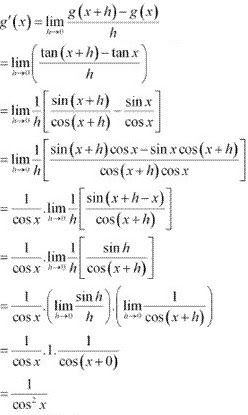= sec2 x  ...(ii)
Therefore, from (i) and (ii), we obtain
f '(x) = (x + cos x)(1 - sec2 x) + (x - tan x)(1 - sin x)
= (x + cos x)(-tan2 x) + (x - tan x)(1 - sin x)
= -tan2 x (x + cos x) + (x - tan x) (1 - sin x)

26. Find the derivative of the following functions (it is to be understood that a, b, c, d, p, q, r and s are fixed non-zero constants and m and n are integers):
Solution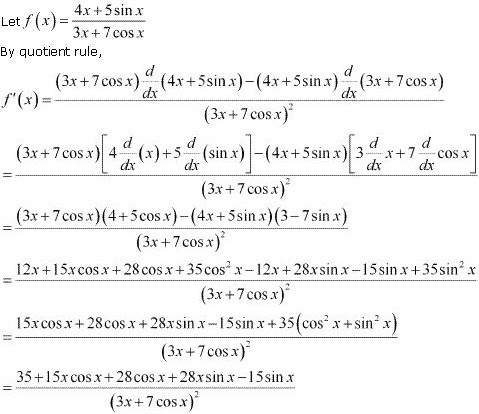27. Find the derivative of the following functions (it is to be understood that a, b, c, d, p, q, r and s are fixed non-zero constants and m and n are integers):
Solution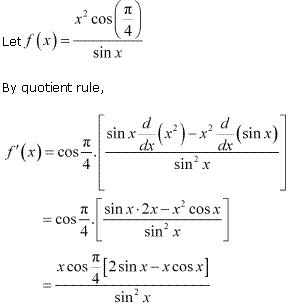28. Find the derivative of the following functions (it is to be understood that a, b, c, d, p, q, r and s are fixed non-zero constants and m and n are integers): x/(1 + tan x)
Solution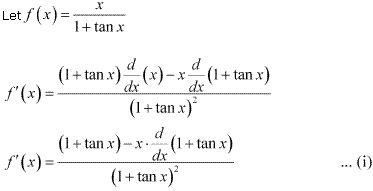Let g(x) = 1 + tan x. Accordingly, g(x + h) = 1 + tan (x + h).
By first principle,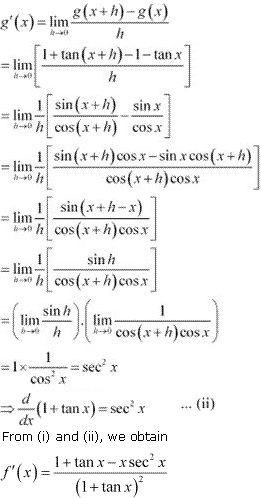29. Find the derivative of the following functions (it is to be understood that a, b, c, d, p, q, r and s are fixed non-zero constants and m and n are integers): (x + sec x) (x – tan x)
Solution
Let f(x) = (x + sec x)(x -tan x)
By product rule,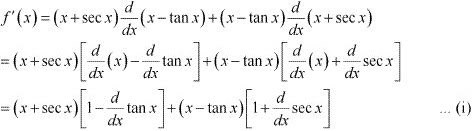Let f1 (x) = tan x, f2 (x)  = sec x
Accordingly, f1 (x + h) = tan (x + h) and f2(x + h) = sec (x + h)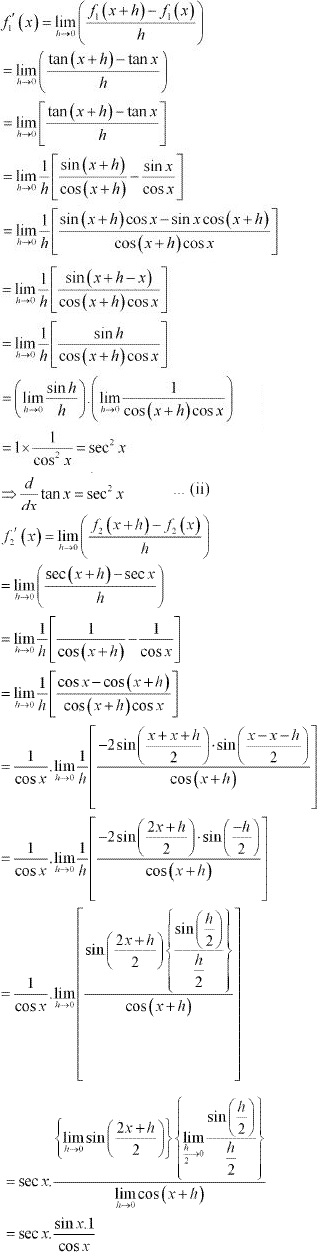⇒ (d/dx) sec x = sec x tan x ...(iii)
From (i), (ii) and (iii), we obtain
f'(x) = (x + sec x) (1 - sec2 x) + (x - tan x)( 1 + sec x tan x)

30. Find the derivative of the following functions (it is to be understood that a, b, c, d, p, q, r and s are fixed non-zero constants and m and n are integers): x/sinn x
Solution
Let f(x) =  x/sinn x
By quotient rule,